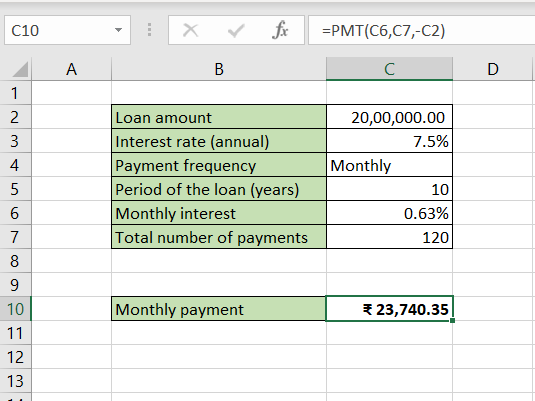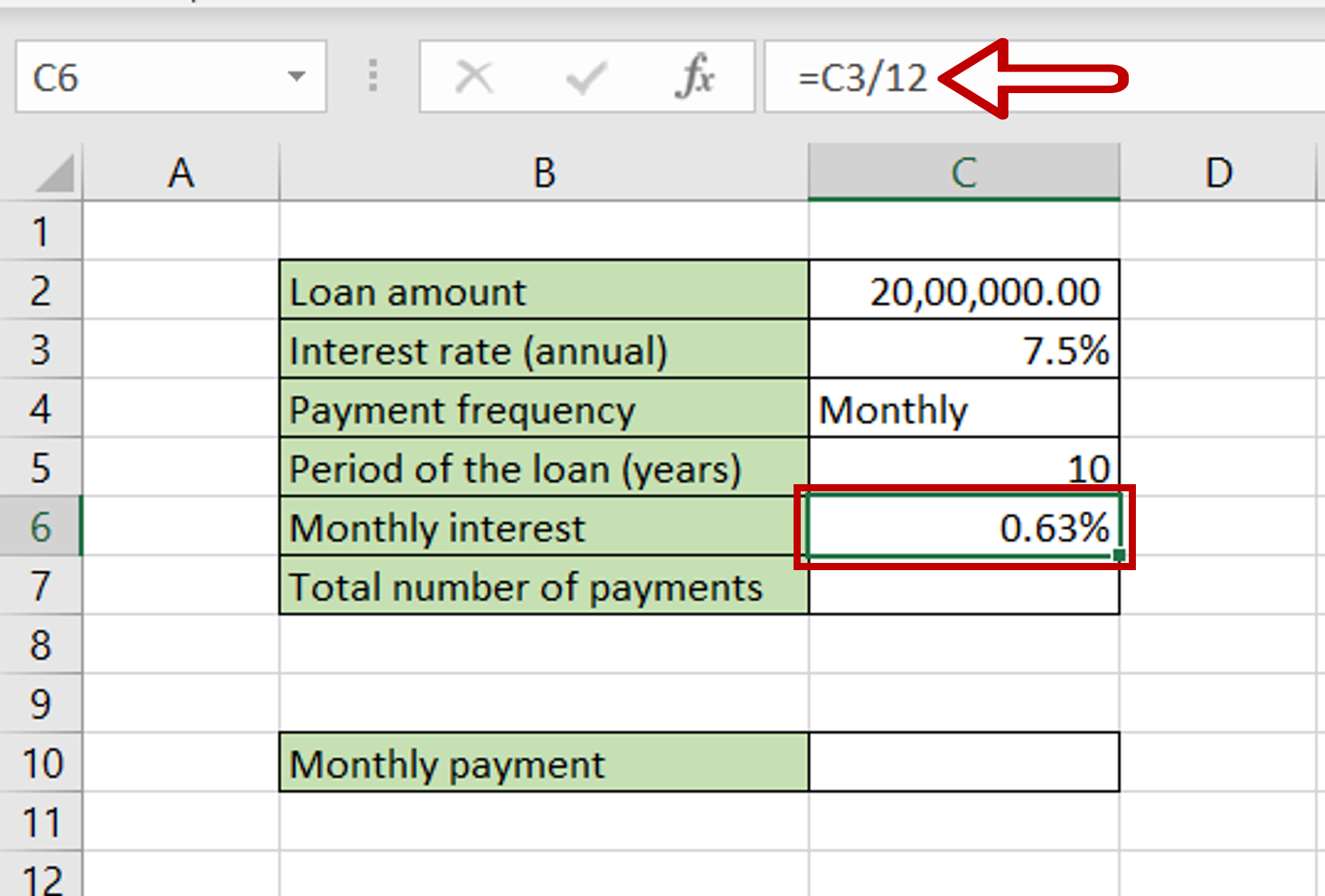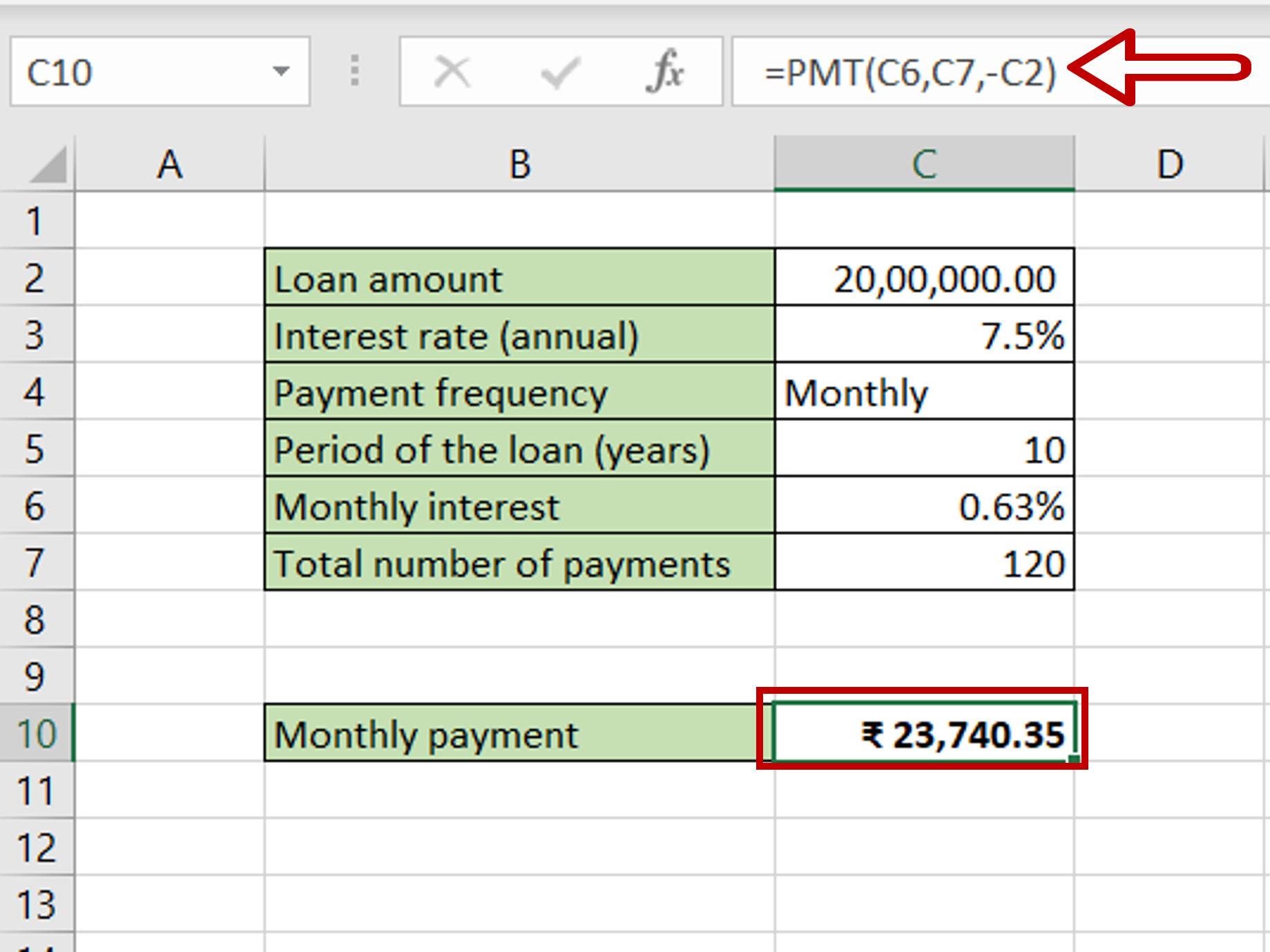# How to calculate monthly payment on a loan in Excel

You can watch a video tutorial here.Excel is frequently used for calculations and supports all basic mathematical operations. Using formulas, you can use Excel to build different types of calculators in which you only need to change the values to get the result. This saves you the problem of doing each step of the calculation repeatedly. For example, you can build a calculator to calculate the monthly payment on a loan based on the loan amount. Using this, you can compare loans from various providers to understand which suits you best. In Excel, you can use the PMT function to compute the monthly payment.

1. PMT() function: this calculates the monthly payment for a loan
a) Syntax: PMT(rate, nper, pv, [fv], [type])
i. rate: the interest rate
ii. nper: the total number of payments
iii. pv: the principal
iv. [fv] optional: remaining cash balance after the last payment is made, by default this is zero
v. [type] optional: specify whether the payment is due at the beginning or end of the loan

In this example, given the basic parameters of the loan, we will compute the inputs for the PMT() function using mathematical operations.

### Step 1 – Find the monthly interest rate– Select the cell for the monthly interest to be displayed
– Type the formula using cell references:
= Interest rate (annual)/12
– Press Enter

### Step 2 – Find the total number of payments– Select the cell for the total number of payments to be displayed
– Type the formula using cell references:
= Period of the loan (years) * 12
Because the payment frequency is monthly, the number is multiplied by 12
– Press Enter

### Step 3 – Create the formula using PMT()– Select the cell for the monthly payment to be displayed
– Type the formula using cell references:
= PMT(Monthly interest, Total number of payments, – Loan amount)
Note: The PMT() function returns a negative number, so a minus sign is put in front of the ‘Loan amount’ to make the result a positive number.
– Press Enter